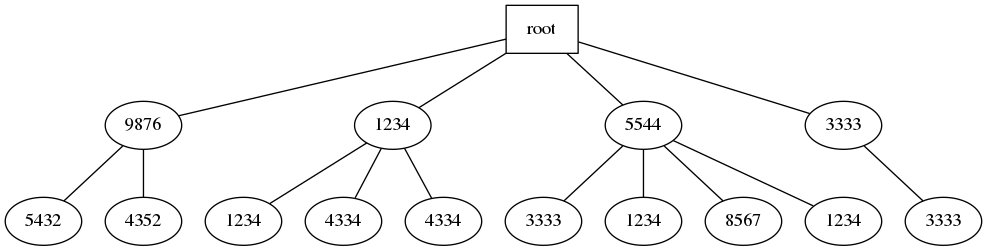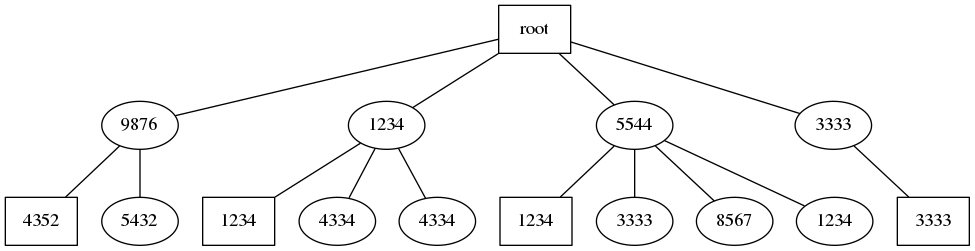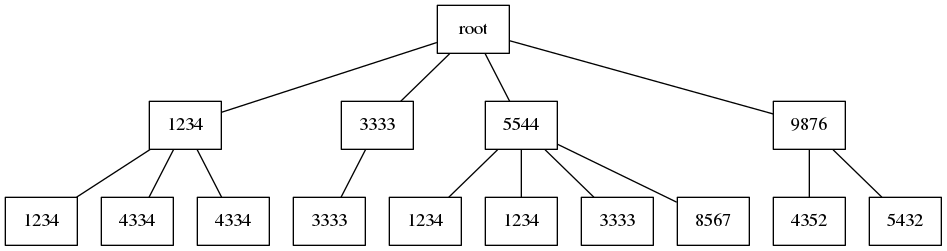Radix sort sorts n w-bit integers by splitting them up into chunks of $\log n$ bits each, and sorting each chunk in linear time. Thus it achieves $O(nw/\log n)$ time.

In 1983 Kirkpatrick and Reisch1 published an algorithm that improves on this. It achieves time that has an exponentially smaller factor next to n:

$O\left(n + n \log\frac{w}{\log n}\right)$

As originally published, the algorithm is deterministic, at the cost of using a huge $\left(\Theta(2^{w/2})\right)$ amount of memory. Instead, it is more practical to combine the idea with universal hashing to get a randomized algorithm with that expected time, and linear space.

## Step 1. Build depth-2 trie.

Suppose we want to sort this list of 10 numbers:

98765432
12341234
55443333
55441234
12344334
55448567
33333333
12344334
55441234
98764352


We split each number into the top half of bits and bottom half (in our case we will take 4 decimal digits each). Then we add the number to the trie as a length-2 path: at the first level we have the more significant bits, in the leaves we have the least significant bits.Note that when building the trie we have to be able to look up nodes at the first level by value, to avoid duplicating them. This is where hashing (and hence randomization) comes in. In the leaves duplicates are OK.

## Step 2. Find the smallest leaf in each subtree.

We find the minimum leaf and make it the first child in each subtree.## Step 3. Sort remaining nodes.

We take all the nodes other than root and the minimum leaves, and sort them recursively.

There are n leaves. We added some number of level-1 nodes, but skip the same number of minimum leaves. Thus the recursive sort also sorts n numbers, with half as many bits each.

Nodes that we need to sort (in breadth-first order):

9876
1234
5544
3333
5432
4334
4334
3333
8567
1234


After sorting:

1234
1234
3333
3333
4334
4334
5432
5544
8567
9876


## Step 4. Sort children edges.

Using the sorted order of nodes computed in the previous step we can reorder all the edges so that they are in sorted order.

We simply detach all the children (except the minimum leaves), and then walk all the nodes in sorted order and re-attach them to their original parent.## Step 5. Walk the sorted trie.

We now simply walk the trie left-to-right and re-combine high bits with low bits to get the final sorted answer:

12341234
12344334
12344334
33333333
55441234
55441234
55443333
55448567
98764352
98765432


## Time complexity

Steps 1, 2, 4, 5 take linear time. Step 3 requires recursive sorting of numbers that are half as long.

If we let $T(n, b)$ be the time to sort n b-bit numbers, we get the recurrence:

$T(n, b) = T(n, \lceil b/2 \rceil) + O(n)$

We stop the recursion once the numbers have at most $\log n$ bits. At that point, we can just sort in linear time by counting each value. Thus:

$T(n, \lfloor \log n \rfloor) = O(n)$

We start with w bits each, and want to get to $\log n$ bits each. Each level of recursion halves the number of bits, so we need $\log (w / \log n)$ levels.

Thus the total time complexity is:

$O\left(n + n \log\frac{w}{\log n}\right)$
1. Kirkpatrick, David, and Stefan Reisch. “Upper bounds for sorting integers on random access machines.” Theoretical Computer Science 28.3 (1983): 263-276.